Question

# design an op amp comparator with the following characteristics v=10v, vd=0.7v and Rf=infinity

design an op amp comparator with the following characteristics v=10v, vd=0.7v and Rf=infinity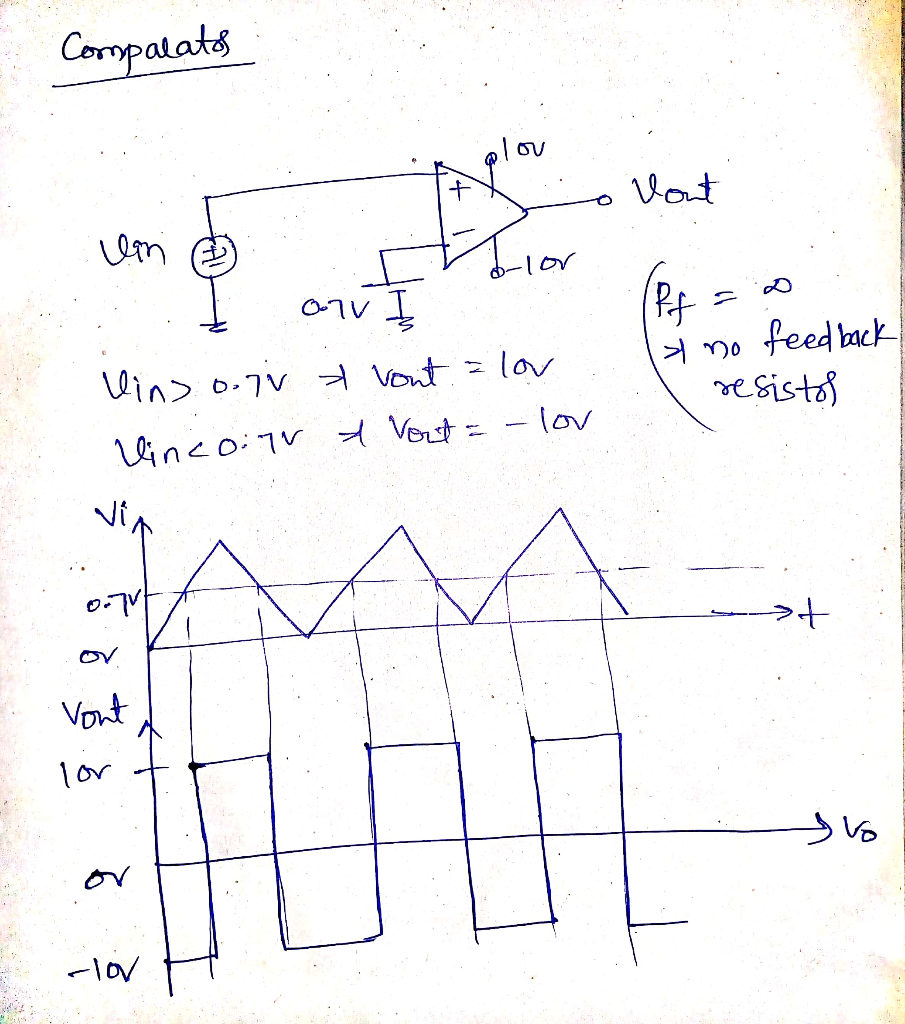#### Earn Coins

Coins can be redeemed for fabulous gifts.

Similar Homework Help Questions
• ### Example Analyze the response of a simple op-amp comparator and Schmitt Trigger of Figure 1 with...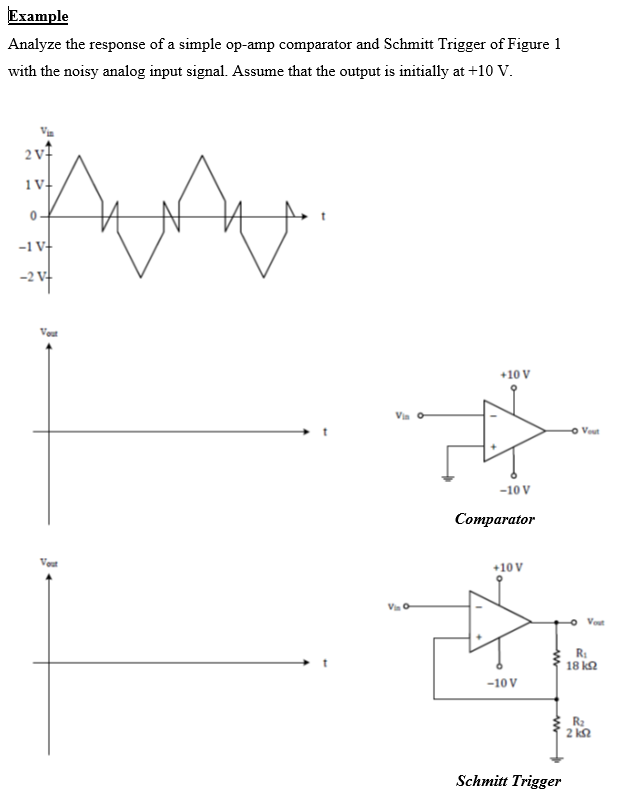Example Analyze the response of a simple op-amp comparator and Schmitt Trigger of Figure 1 with the noisy analog input signal. Assume that the output is initially at +10 V. 2v+ 1VH hun 0 -1 VH -2v7 +10 V "A - Vou -10 V Comparator Vou +10 V Vous R: 18k2 -10V R2 2 ΚΩ Schmitt Trigger

• ### Assume the op-amp below is used as a comparator circuit and that the op-amp is powered...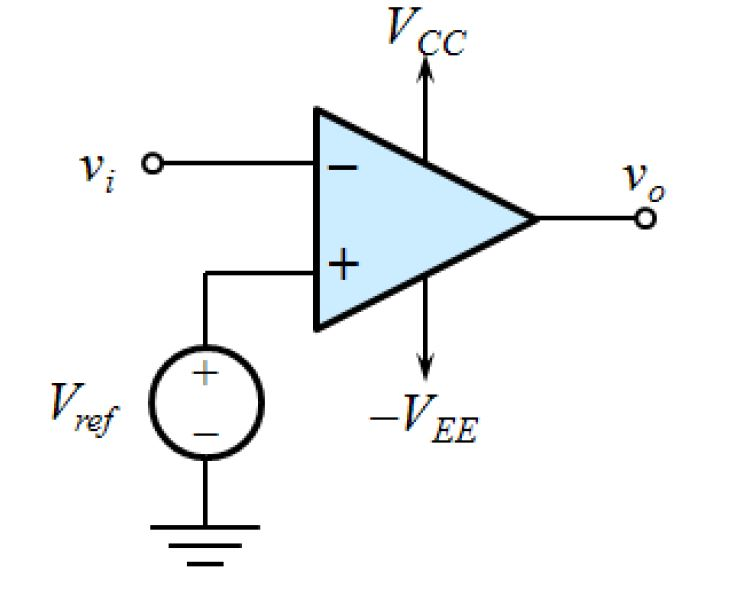Assume the op-amp below is used as a comparator circuit and that the op-amp is powered by a bipolar +/- 15 V power supply. If the reference voltage Vref = -9 V and the input voltage is VI = 12 V, determine the output voltage, VO, of the op-amp. Assume the open-loop gain, A, is very large and consider saturation effects. Include units in your answer. VCC Vi V. + + Vref EE

• ### 1. Use supply voltage +/- 15V and design a comparator op-amp circuit where the output becomes...

1. Use supply voltage +/- 15V and design a comparator op-amp circuit where the output becomes maximum positive when the input is higher then 5V. 2. Sketch the output voltage relative to the input voltage of the circuit. 3. How can we get maximum negative output voltage when the input voltage is bigger then 5V?

• ### Q 1. An op amp with an open-loop gain of 6 x 105 and Vcc =...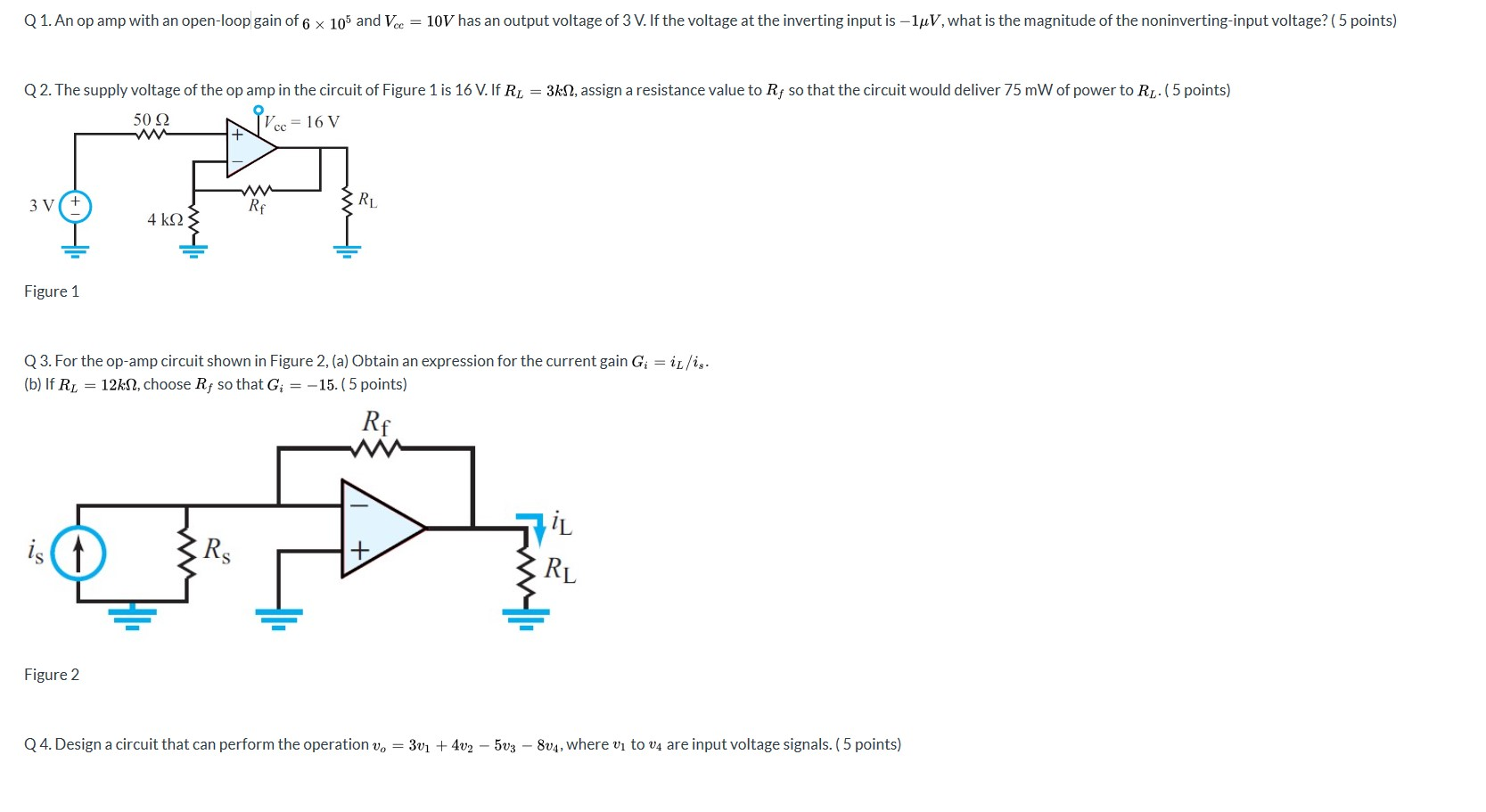Q 1. An op amp with an open-loop gain of 6 x 105 and Vcc = 10V has an output voltage of 3 V. If the voltage at the inverting input is -luV, what is the magnitude of the noninverting-input voltage? (5 points) Q2. The supply voltage of the op amp in the circuit of Figure 1 is 16 V. If R = 3kN, assign a resistance value to Rf so that the circuit would deliver 75 mW of power...

• ### pts) Consider the following Op Amp Circuit, use Op Amp rules to calculate vo vi and...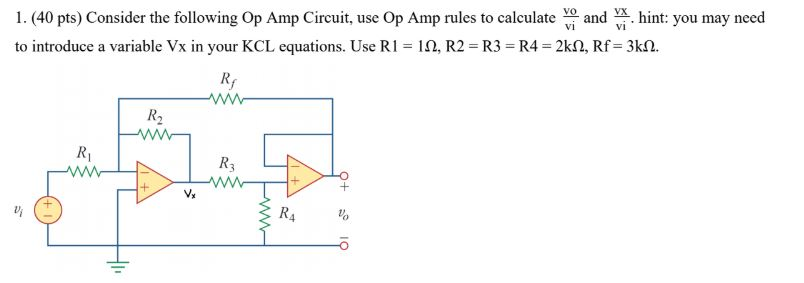pts) Consider the following Op Amp Circuit, use Op Amp rules to calculate vo vi and vx vi . hint: you may need to introduce a variable Vx in your KCL equations. Use R1 = 1Ω, R2 = R3 = R4 = 2kΩ, Rf = 3kΩ. 1. (40 pts) Consider the following Op Amp Circuit, use Op Amp rules to calculated and hint: you may need to introduce a variable Vx in your KCL equations. Use R1 = 12, R2...

• ### 7. Design an Op Amp circuit that solves the following first order differential equation for v(t):...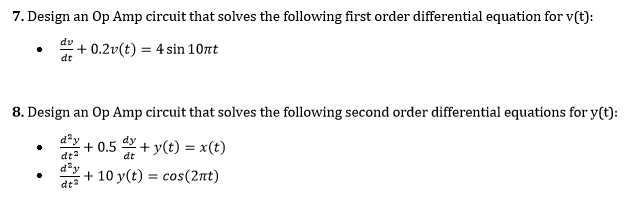7. Design an Op Amp circuit that solves the following first order differential equation for v(t): dv dt 8. Design an Op Amp circuit that solves the following second order differential equations for y(t): 습+10 y(t) = cos(21t) · dt?

• ### Problem 7 (CLO 3, 4, 11 - Ideal Op-Amps, Design): We will now design an Op-Amp...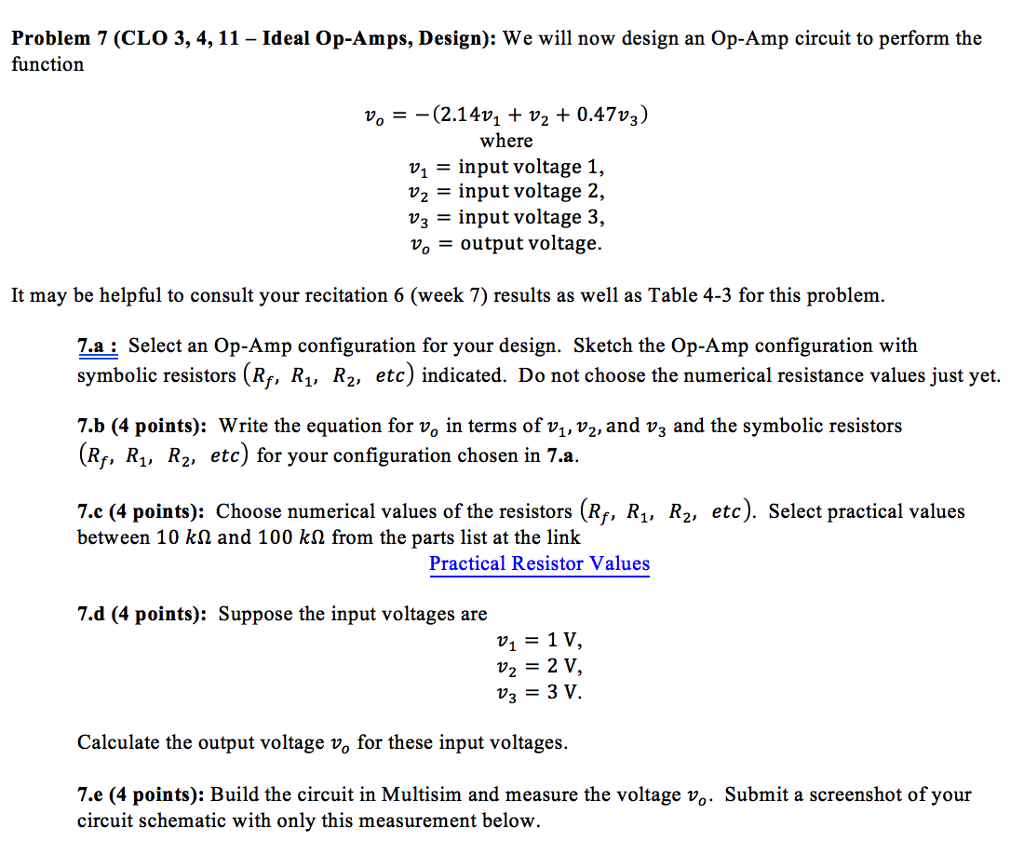Problem 7 (CLO 3, 4, 11 - Ideal Op-Amps, Design): We will now design an Op-Amp circuit to perform the function where v1 - input voltage 1, input voltage 2, = input voltage 3, Vo = output voltage. It may be helpful to consult your recitation 6 (week 7) results as well as Table 4-3 for this problem. 7.a: Select an Op-Amp configuration for your design. Sketch the Op-Amp configuration with symbolic resistors (Rf, Ri, R2, etc) indicated. Do not...

• ### Design an inverting op amp circuit with gain-12. Resistors available are 10K, 12K, 100K, 120K, and...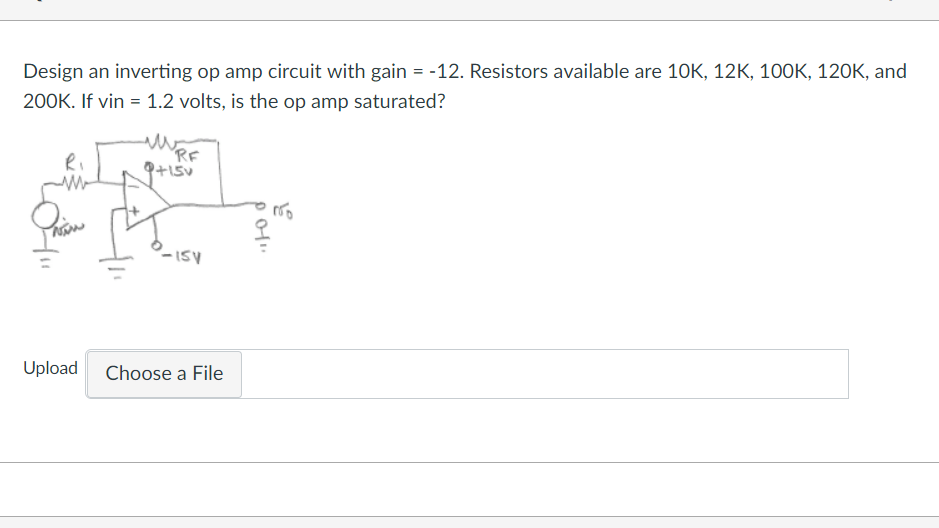Design an inverting op amp circuit with gain-12. Resistors available are 10K, 12K, 100K, 120K, and 200K. If vin 1.2 volts, is the op amp saturated? RF ? IS Upload Choose a File

• ### 1- Design a lossy integrator op-amp circuit using op-amp 741. First, derive an expression for - V...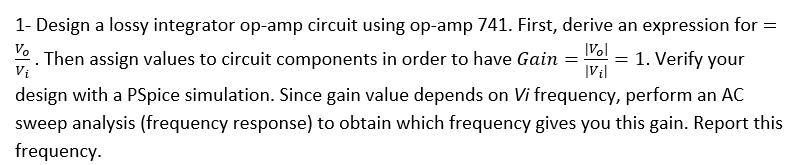UPLOAD PSPICE SIMULATION TO VERIFY CALCULATIONS 1- Design a lossy integrator op-amp circuit using op-amp 741. First, derive an expression for - Vo Then assign values to circuit components in order to have Gai 1. Verify your design with a PSpice simulation. Since gain value depends on Vi frequency, perform an AC sweep analysis (frequency response) to obtain which frequency gives you this gain. Report this trequency. IVil 1- Design a lossy integrator op-amp circuit using op-amp 741. First, derive...

• ### V in Figure 1: Op-amp circuit 1 V in V out Figure 2: Op-amp circuit 2 1 Assume that the op amp is ideal, and that it ha...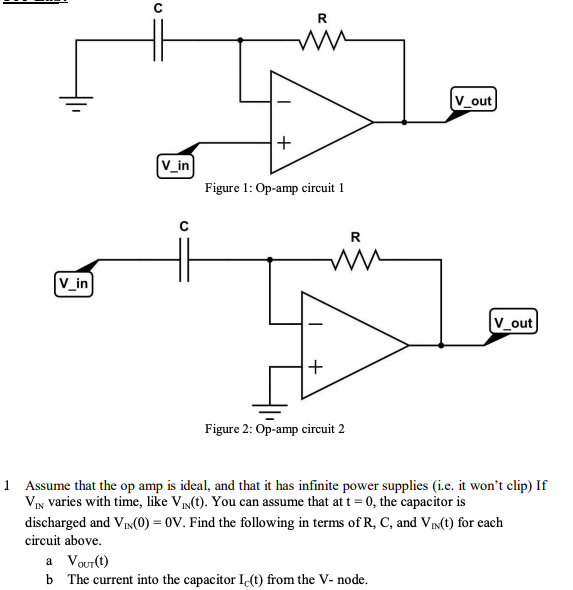V in Figure 1: Op-amp circuit 1 V in V out Figure 2: Op-amp circuit 2 1 Assume that the op amp is ideal, and that it has infinite power supplies (i.e. it won't clip) If VIN varies with time, like ViI(t). You can assume that at t-0, the capacitor is discharged and VIN(0)-OV. Find the following in terms of R, C, and VIn(t) for each circuit above. a Vourt) b The current into the capacitor Ic() from the V-...